Get instant live expert help with Excel or Google Sheets“My Excelchat expert helped me in less than 20 minutes, saving me what would have been 5 hours of work!”

#### Post your problem and you’ll get Expert help in seconds.

Your message must be at least 40 characters
Our professional Expert are available now. Your privacy is guaranteed.

# Basic overtime calculation formula

We can use a set of formulas to calculate the pay associated with workers working overtime. The steps below will guide all levels of excel users through the process.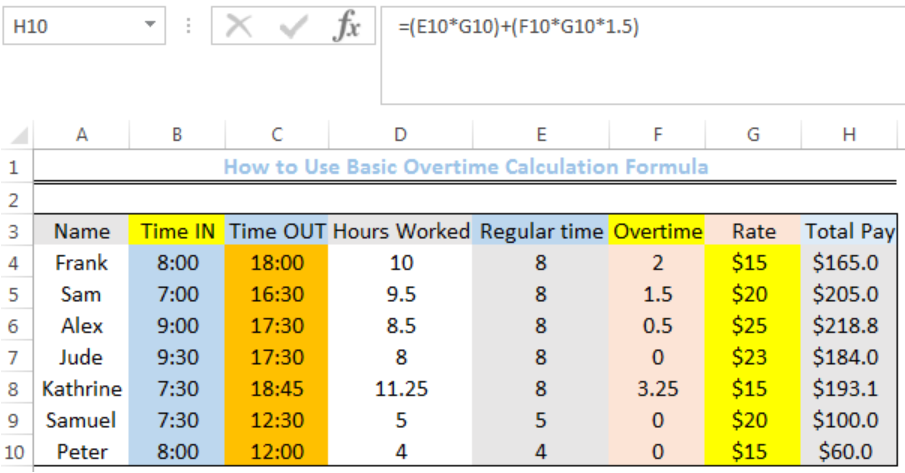Figure 1: How to Use Basic overtime calculation formula

## General Formula

`=(regular time*rate) + (overtime*rate*1.5)`

## Formula

Total pay for overtime: `=(E4*G4)+(F4*G4*1.5)`

Total hours worked: `=(C4-B4)*24`

Regular time formula: `=MIN(8,D4)`

Overtime: `=D4-E4`

## Setting up the Data

• We will set up the data by inputting the Names, Time IN, Time OUT, and RATE for 7 employees in Column A, Column B, Column C, and Column G respectively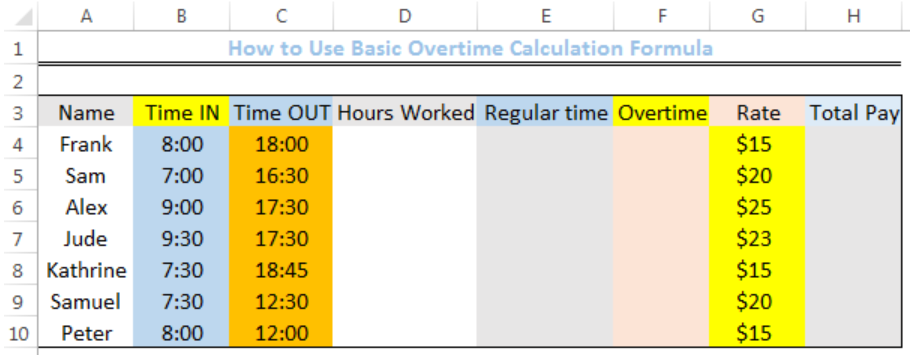Figure 2: Setting up the Data

## Calculating the Hours Worked

Excel calculates time as fractions of a day. Hence, 12;00 PM is 12/24 = 0.5, 3:00 AM is 3/24 = 0.125, and so on. To ensure that we do not encounter problems, we will convert the time from its native unit to decimal hours in Column D.

• We will click on Cell D4
• We will insert the formula below into the cell
`=(C4-B4)*24`
• We will press the enter key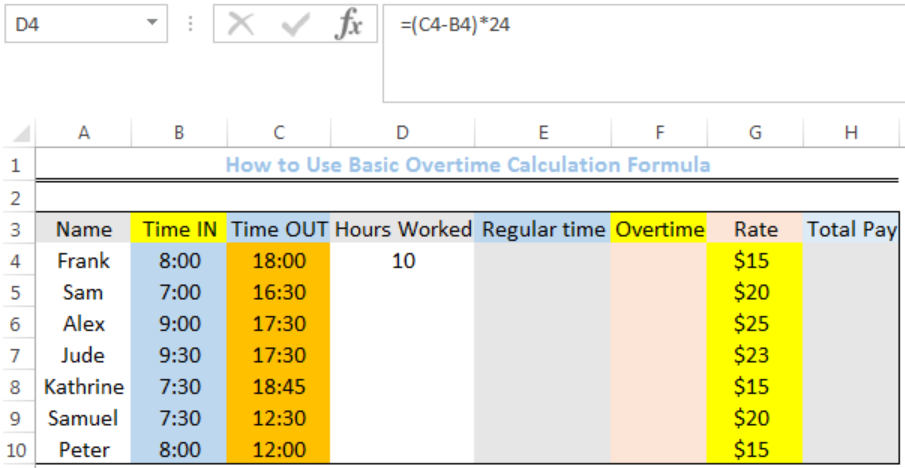Figure 3: Hours worked for Cell D4

• We will click on Cell D4 again
• We will double click on the fill handle tool which is the small plus sign you see at the bottom right of Cell D4. Select and drag down to copy the formula to other cells.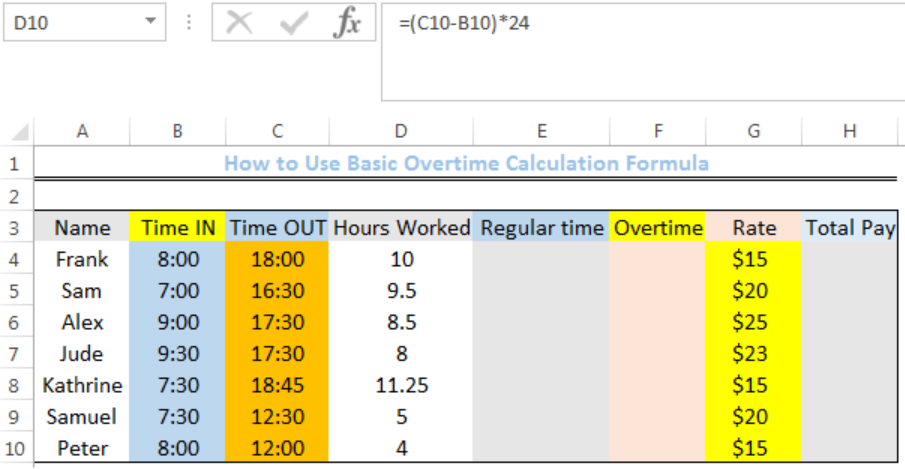Figure 4: Hours worked in Column D

## Calculating the Regular Work Time

• We will click on Cell E4
• We will insert the formula below into the cell
`=MIN(8,D4)`
• We will press the enter key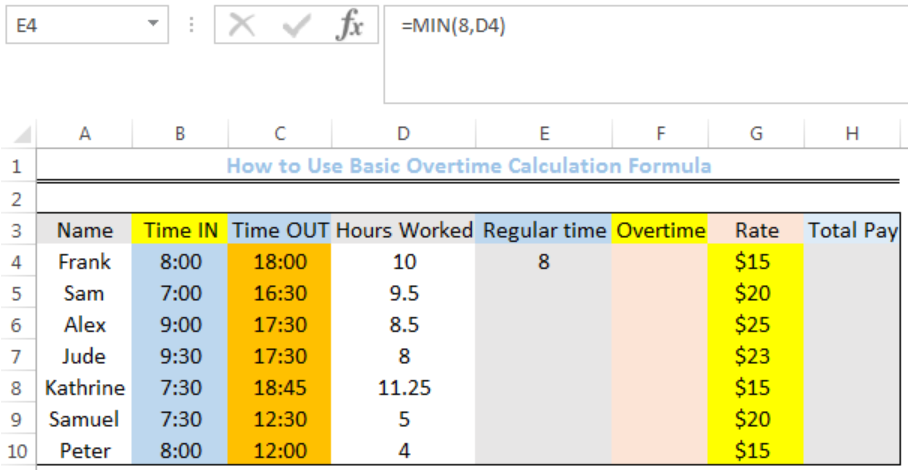Figure 5: Regular time for Cell E4

• We will click on Cell E4 again
• We will double click on the fill handle tool which is the small plus sign you see at the bottom right of Cell E4. Select and drag down to copy the formula to other cells.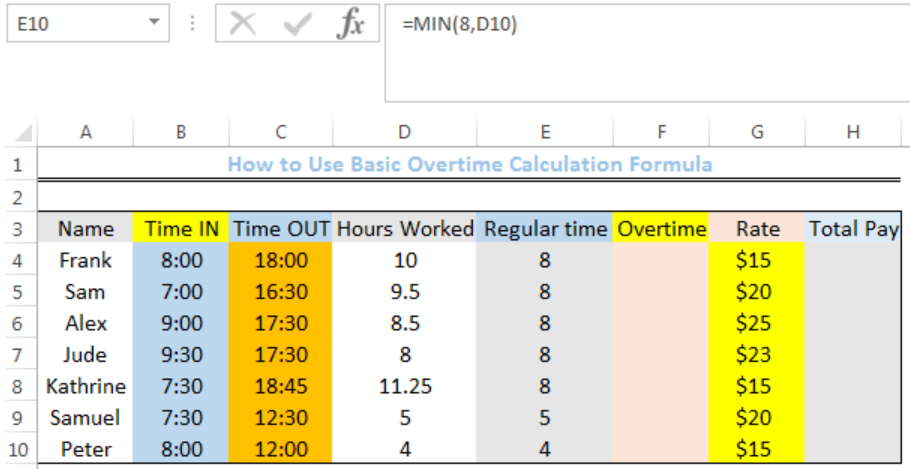Figure 6: Regular time for Column E

## Calculating the Overtime

• We will click on Cell F4
• We will insert the formula below into the cell
`=D4-E4`
• We will press the enter key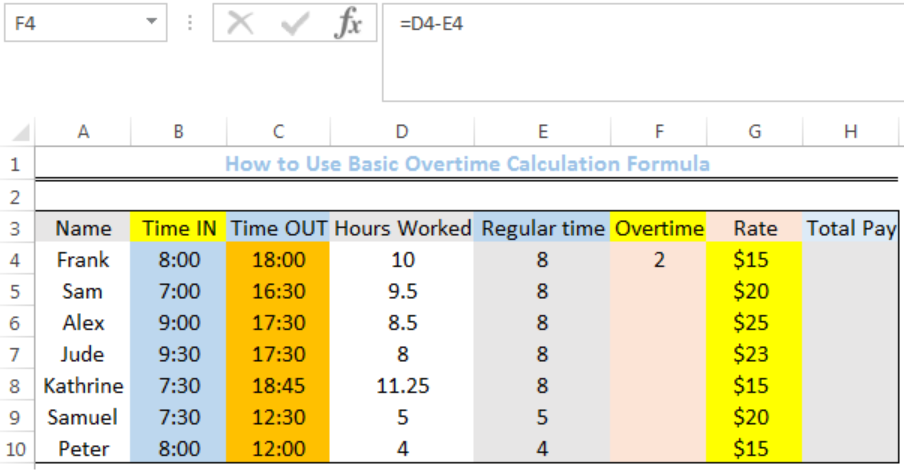Figure 7: Overtime for Cell F4

• We will click on Cell F4 again
• We will double click on the fill handle tool which is the small plus sign you see at the bottom right of Cell F4. Select and drag down to copy the formula to other cells.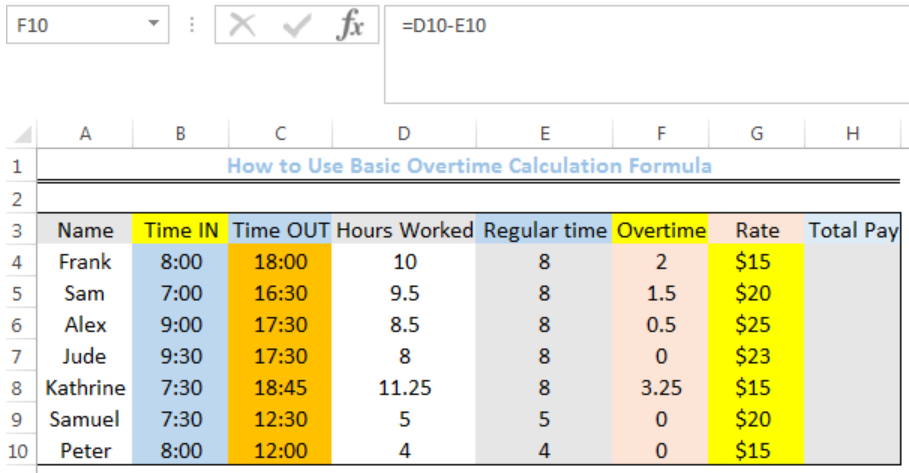Figure 8: Overtime for Column F

## Calculating the Total Pay

• We will click on Cell H4
• We will insert the formula below into the cell
`=(E4*G4)+(F4*G4*1.5)`
• We will press the enter key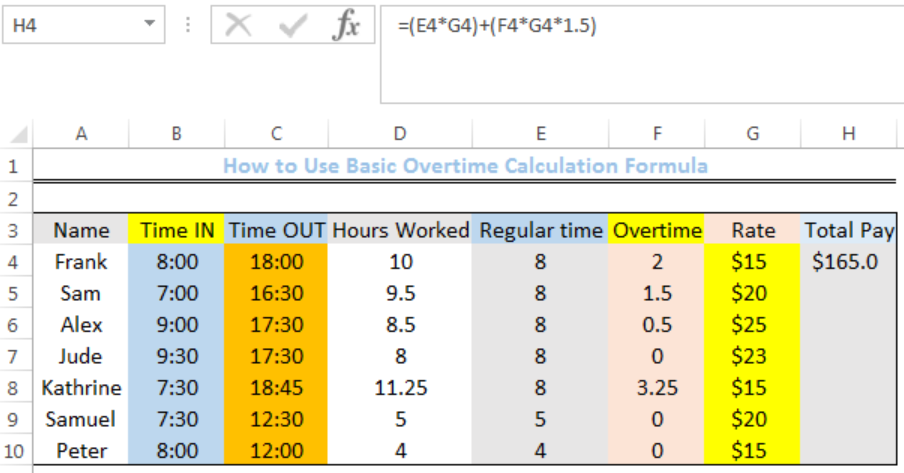Figure 9: Total Pay for Cell H4

• We will click on Cell H4 again
• We will double click on the fill handle tool which is the small plus sign you see at the bottom right of Cell H4. Select and drag down to copy the formula to other cells.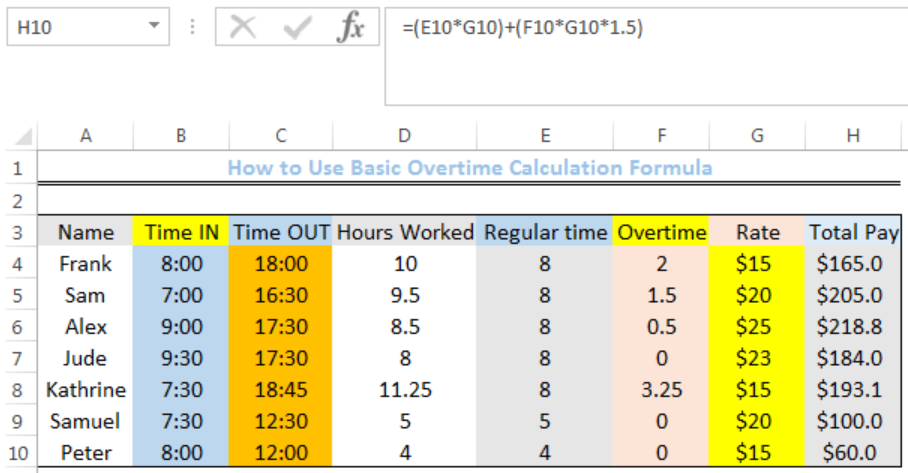Figure 10: Total Pay for Column H

## Explanation

`=(E4*G4)+(F4*G4*1.5)`

`=(regular time*rate) + (overtime*rate*1.5)`

In this formula, the overtime pay is 1.5 times the normal pay. When overtime is 0, the right side of the formula (overtime*rate*1.5) will be zero and the employee will receive only the normal pay.

## Note

• Ensure that you are working on an excel sheet that doesn’t have a prior formula.

## Instant Connection to an Expert through our Excelchat Service

Most of the time, the problem you will need to solve will be more complex than a simple application of a formula or function. If you want to save hours of research and frustration, try our live Excelchat service! Our Excel Experts are available 24/7 to answer any Excel question you may have. We guarantee a connection within 30 seconds and a customized solution within 20 minutes.

### Did this post not answer your question? Get a solution from connecting with the expert.Another blog reader asked this question today on Excelchat:## Subscribe to Excelchat.coAnother blog reader asked this question today on Excelchat: